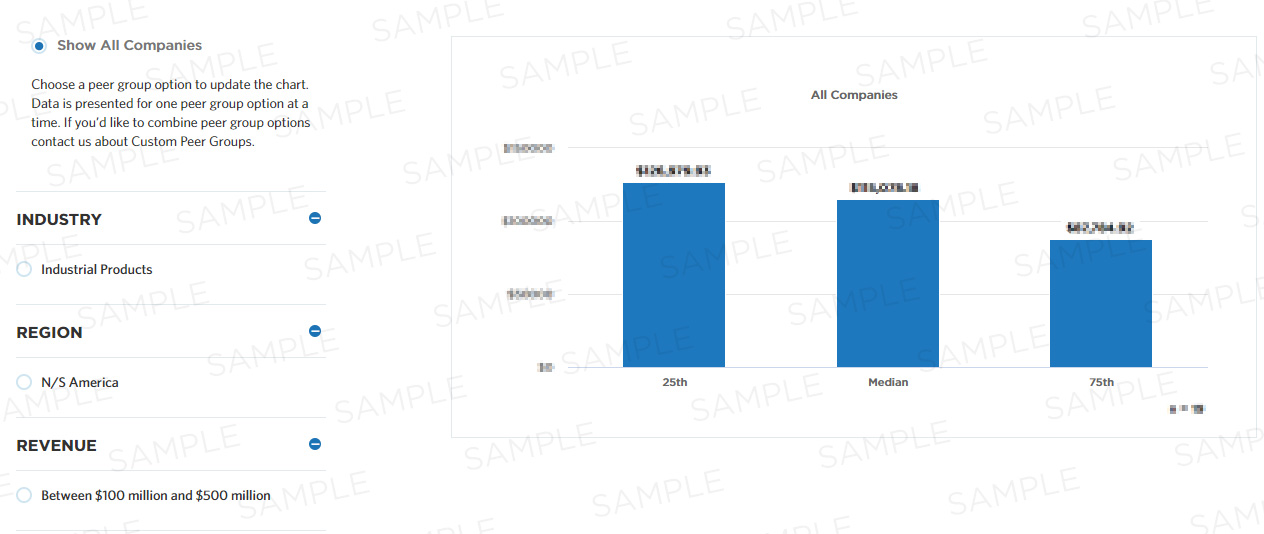# Forecast accuracy one planning period prior to production run

Forecast accuracy compares forecasted demand to actual demand. This measure calculates it for products and/or families for markets/distribution channels one planning period prior to production run. In this case, forecast accuracy = 1 - the absolute value of the quantity (actual number of units - forecast number of units) divided by the actual number of units where: forecast number of units = the units forecasted for the production run immediately following the planning period actual number of units = the units actually produced for the production run immediately following the planning period. This measure is part of a set of Process Efficiency measures that help companies optimize the performance of their "measure forecast accuracy" process by minimizing waste and refining resource consumption.

## Benchmark Data

Measure Category:
Process Efficiency
Measure ID:
100737
Total Sample Size:
1,035 All Companies
Performers:
25th
-
Median
-
75th
-
Key Performance
Indicator:
No## Compute this Measure

Units for this measure are percent.

`Forecast accuracy one planning period prior to production run`

## Key Terms

#### Forecast accuracy one planning period prior to production run

Forecast accuracy one planning period prior to production run is calculated for products and/or families for markets/distribution channels in unit measurement.

Forecast accuracy = 1- the absolute value of the quantity (actual number of units - forecast number of units) divided by the actual number of units where:

forecast number of units = The units forecasted for the production run immediately following the planning period
actual number of units = the units actually produced for the production run immediately following the planning period

#### Process efficiency

Process efficiency represents how well a process converts its inputs into outputs. A process that converts 100% of the inputs into outputs without waste is more efficient than one that converts a similar amount of inputs into fewer outputs.